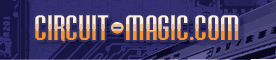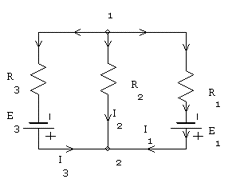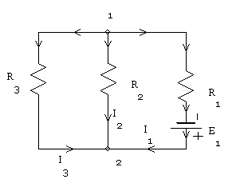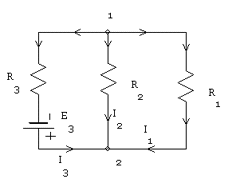Superposition theorem. Using Circuit Magic to analyse any electrical circuitCircuit analysis Tutorial
AKNM Circuit Magic- circuit analysis software
Superposition theorem

In a network with multiple voltage sources, the current in any branch is the sum of the currents which would flow in that branch due to each voltage source acting alone with all other voltage sources replaced by their internal impedances.

The goal of folowing text is to check superposition theorem.

Step 1. Construct following circuit using Circuit Magic then run Node Voltage Analysis. (popular circuits analysis technique). You can alsocalculate currents using other techniquesElectrical scheme

Inital variables
R
2=10Ohms; R1=10Ohms; R3=10Ohms;
E
1=3V; E3=4V;
Solution
V
1·G11=I11
G11=1/R1+1/R2+1/R3=0,3
I
11=-E1/R1-E3/R3=-0,7
0,3V1=-0,7
V
1=-2,3333
V
2=0
I
1=(V1-V2+E1)/R1=0,0666667
I
2=(V1-V2)/R2=-0,233333
I
3=(V1-V2+E3)/R3=0,166667

These values are used to check currents determined from superposition theorem

Step 2. Remove a voltage source from the third branch then run Node Voltage Analysis.Electrical scheme
Inital variables
R
2=10Ohms; R1=10Ohms; R3=10Ohms;
E
1=3V;
Solution
V
1·G11=I11
G11=1/R1+1/R2+1/R3=0,3
I
11=-E1/R1=-0,3
0,3V1=-0,3
V
1=-1
V
2=0
I
1(1)=(V1-V2+E1)/R1=0,2
I
2(1)=(V1-V2)/R2=-0,1
I
3(1)=(V1-V2)/R3=-0,1

These values are used to determine current from superposition theorem.

Step 3. Remove a voltage source from the first branch then run Node Voltage Analysis.Electrical scheme
Inital variables
R
2=10Ohms; R1=10Ohms; R3=10Ohms;
E
3=4V;
Solution
V
1·G11=I11
G11=1/R1+1/R2+1/R3=0,3
I
11=-E3/R3=-0,4
0,3V1=-0,4
V
1=-1,3333
V
2=0
I
1(2)=(V1-V2)/R1=-0,133333
I
2(2)=(V1-V2)/R2=-0,133333
I
3(2)=(V1-V2+E3)/R3=0,266667

Superposition theorem checking

I1=I1(1)+I1(2)=0,2-0,133333=0,0666666
I2=I2(1)+I2(2)=-0,1-0,133333=-0,233333
I3=I3(1)+I3(2)==-0,1+0,266667=0,166667